Subsections

# Equipartition Theorem

Suppose we have l degrees of freedom (generalized coordinates) q1, q2,..., ql and corresponding momenta p1, p2,..., pl, and all q's are close to their equilibrium values (take them to be zero, and qi will be deviations from equilibrium positions):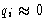. We want to calculate thermodynamics of such a system.

Example:
A simple model of a crystal--masses m on springs with coefficients k. All masses sit near their equilibrium positions! We have 3N degrees of freedom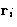, i=1,..., N.Kinetic energy: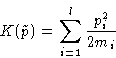Potential energy: let's expand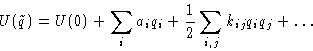The first term does not matter--we'll drop it! The second term is zero (we are at equilibrium!)
Harmonic approximation:
cubic and higher terms are small, and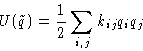(1)

In harmonic approximation both potential and kinetic energy are quadratic forms of coordinates and momenta.

Example:
For our crystal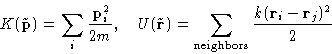Expanding U, we recover quadratic form (1)

## Partition Function and Thermodynamic Functions

### Partition function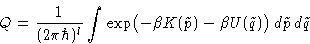(2)
with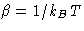Note:
In this lecture we use partition function instead of configuration integral and kB instead of k for Boltzmann constant (why?)

### Change of Variables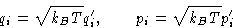New energy:New differentials: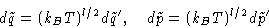Classical partition function: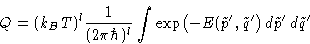The integral does not depend on temperature!

### Thermodynamics

Free energy: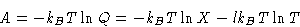with X not dependent on T. Entropy, energy and heat capacity: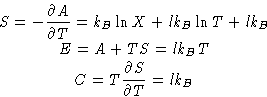We have l degrees of freedom, and energy is lkBT: one kBT for each degree of freedom!

What happens, if for some qi potential energy is zero (rotational and translational degrees of freedom)? Then it contributes kBT/2.

## Equipartition Theorem and Its Applications

In harmonic approximation for classical systems each degree of freedom contributes kBT to energy and kB to heat capacity unless potential energy is identically zero. In the latter case it contributes kBT/2 and kB/2 correspondingly.

Examples:

1.
Monoatomic ideal gas. 3N degrees of freedom. Energy 3kBTN/2, heat capacity 3kBN/2
2.
Diatomic ideal gas, no vibrations. 5N degrees of freedom, energy 5kBNT/2, heat capacity 5kBN/2
3.
Diatomic ideal gas, vibration is allowed. 6N degrees of freedom, one of them vibrational. Energy 7kBNT/2, heat capacity 7kBN/2
4.
Solid body. 3N vibrational degrees of freedom. Energy 3kBNT, heat capacity 3kBN. Molar heat capacity 3R (Dulong & Petit law).

## What's Wrong With Equipartition Theorem?

Equipartition theorem is fine--except sometimes it does not work!

1.
It predicts different results for gases whether we allow vibration or not--but should not it be always allowed?
2.
It predicts heat capacity 3kBN for solids. This is right--at high temperatures. For diamond high'' means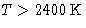, sometimes it is 100-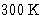.

Reason:
Vibrations are not classical. They are quantum. We need to include quantum mechanics to describe them properly!Next: Harmonic Oscillator Up: Solid State Previous: Solid State

© 1997 Boris Veytsman and Michael Kotelyanskii
Wed Oct 1 00:45:35 EDT 1997